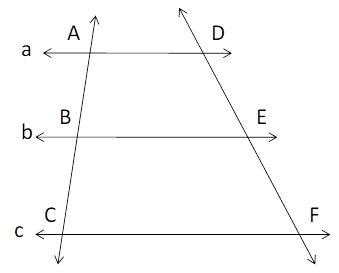Chapter 4.4, Problem 26EElementary Geometry For College St...

7th Edition
Alexander + 2 others
ISBN: 9781337614085

Solutions

Chapter
SectionElementary Geometry For College St...

7th Edition
Alexander + 2 others
ISBN: 9781337614085
Textbook Problem

In the figure, a | | b | | c and B is the midpoint of A C ¯ . If A B = 2 x + 3 y , B C = x + y + 7 and D E = 2 x + 3 y + 3 and E F = 5 x − y + 2 , Find x         a n d           y .Exercises 25, 26

To determine

To Find:

The value of xandy.

Explanation

The given diagram is

Given: a||b||c and B is the midpoint of AC¯.

Also, AB=2x+3y, BC=x+y+7 and DE=2x+3y+3 and EF=5xy+2.

We know that, when three or more parallel lines intercept congruent line segments on one transversal, then they intercept congruent line segments on any transversal.

Therefore, ABBCandDEEF.

Now, 2x+3y=x+y+7.

It becomes 2x+3yxy=7.

Then x+2y=7.

Therefore, x=72y

Still sussing out bartleby?

Check out a sample textbook solution.

See a sample solution

The Solution to Your Study Problems

Bartleby provides explanations to thousands of textbook problems written by our experts, many with advanced degrees!

Get Started

Define a Type I error and a Type Il error and explain the consequences of each.

Statistics for The Behavioral Sciences (MindTap Course List)

Find the medians of the sets of scores in Problems 13-16. 13. 1, 3, 6, 7, 5

Mathematical Applications for the Management, Life, and Social Sciences

Sometimes, Always, or Never: dx equals the area between y = f(x), the x-axis, x = a, and x = b.

Study Guide for Stewart's Single Variable Calculus: Early Transcendentals, 8th

The area of the region bounded by , , and r = sec θ is:

Study Guide for Stewart's Multivariable Calculus, 8th